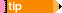Faking Dependencies Faking Dependencies Faking Dependencies Faking Dependencies Faking Dependencies Faking Dependencies Faking Dependencies Faking Dependencies Faking Dependencies Faking Dependencies Faking Dependencies Faking Dependencies Faking Dependencies Faking Dependencies Faking Dependencies Faking Dependencies Faking Dependencies Faking Dependencies Faking Dependencies Faking Dependencies Faking Dependencies

### Faking Dependencies

With a single method, Typemock Isolator creates an object and fakes all the dependencies in its constructor. This approach saves you time and lines of code. In addition, it creates a more robust test. If the constructor changes, dependencies are added or removed, the test remains the same.If there are primitives, structs, or strings that are also accepted by the constructor, they are initialized to their base values.

#### When to Use

When you want to fake all arguments of the constructor.

#### Syntax

```C#

T real = Isolate.Fake.Dependencies<T>()
Isolate.GetFake<F>(real);
```

```VB

Dim real = Isolate.Fake.Dependencies(Of T)()
Isolate.GetFake(Of F)(real)
```

#### Samples

##### Sample 1: Faking All Dependencies of the Type

The following sample shows how to fake all dependencies of the type as follows:

T real = Isolate.Fake.Dependencies<T>()

The constructor of T is called with authomatically created faked objects as parameters for reference types and default values for value types. In the example, the call var real = Isolate.Fake.Dependencies<ClassUnderTest>() is equal to:

var dependency = Isolate.Fake.Instance<Dependency>();
var dependency2 = Isolate.Fake.Instance<Dependency2>();
var real = new ClassUnderTest(0, dependency, dependency2);

var fake = Isolate.GetFake<F>(real)

Because faked objects that where transferred as parameters to the constructor were created authomatically, there is no no reference to these objects. The call will return an authomatically created parameter object of type F. In the example, the call var fake = Isolate.GetFake<Dependency>(real) will return an authomatically created faked object of type Dependency (dependency). This is required if you want to make some changes in the behavior of the dependency. For example:

var fake = Isolate.GetFake<Dependency>(real);
Isolate.WhenCalled(() => fake.SomeMethod()).WillReturn(3);

```C#

[TestMethod]
public void FakeAllDependencies_ChangeBehavior()
{
var real = Isolate.Fake.Dependencies<ClassUnderTest> ();
var fake = Isolate.GetFake<Dependency> (real);
Isolate.WhenCalled(() => fake.Multiplier).WillReturn(2);

var result = real.Calculate(1, 2);
Assert.AreEqual(6, result);
}

public class ClassUnderTest
{
private Dependency2 d2;
private Dependency d1;

public ClassUnderTest(int additional, Dependency2 d2, Dependency d1)
{
this.d2 = d2;
this.d1 = d1;
}

public int Calculate(int a, int b)
{
d2.Check();
}
}
```

```VB
<TestMethod()>
Public Sub FakeAllDependencies_ChangeBehavior()
Dim real = Isolate.Fake.Dependencies(Of ClassUnderTest)()
Dim fake = Isolate.GetFake(Of Dependency)(real)
Isolate.WhenCalled(Function() fake.Multiplier).WillReturn(2)

Dim result = real.Calculate(1, 2)
Assert.AreEqual(6, result)
End Sub

Public Class ClassUnderTest
Private d2 As Dependency2
Private d1 As Dependency

Public Sub New(additional As Integer, d2 As Dependency2, d1 As Dependency)
Me.d2 = d2
Me.d1 = d1
End Sub

Public Function Calculate(a As Integer, b As Integer) As Integer
d2.Check()
Return (a + b) * d1.Multiplier + additional
End Function
End Class
```

##### Sample 2: Passing Specific Arguments to the Constructor

To pass arguments other than auto-fakes, pass them to the Fake.Dependencies() method. Typemock Isolator will try to match the type of the argument that you pass in the constructor and will swap them. This means that you do not need to enter the position of each argument, which protects you from heavy refactoring when changing the dependencies.You can set up a fake behavior before calling the constructor and then pass these fakes through Fake.Dependencies().To keep the interface simple, the Fake.Dependency() method works if the constructor does not accept multiple arguments of the same type (non-primitives or strings). If the constructor accepts multiple arguments of the same type, create fakes using the Fake.Instance() method and pass the arguments as an argument list.

```C#

[TestMethod]
public void FakeAllDependencies_OverrideArguments()
{
var realDependency = new Dependency();
var real = Isolate.Fake.Dependencies<ClassUnderTest>(realDependency, 4);

var result = real.Calculate(1, 2);
Assert.AreEqual(4, result);
}
```

```VB
<TestMethod()>
Public Sub FakeAllDependencies_OverrideArguments()
Dim real = New Dependency()
Dim fake = Isolate.Fake.Dependencies(Of ClassUnderTest)(real, 4)

Dim result = fake.Calculate(1, 2)
Assert.AreEqual(4, result)
End Sub
```